# RD Sharma Solutions Class 12 Straight Line In Space Exercise 28.4

RD Sharma Solutions for Class 12 Maths Chapter 28 Straight Line in Space Exercise 28.4, is provided here. Students who wish to strengthen their knowledge and have a strong grip over the subject can refer to RD Sharma Solutions. Solutions are designed in a simple language by our experts to meet the needs of all students. Here, the solutions to this exercise are provided in pdf format, which can be downloaded easily from the links given below.

## Download PDF of Rd Sharma Solution for Class 12 Maths Chapter 28 Exercise 4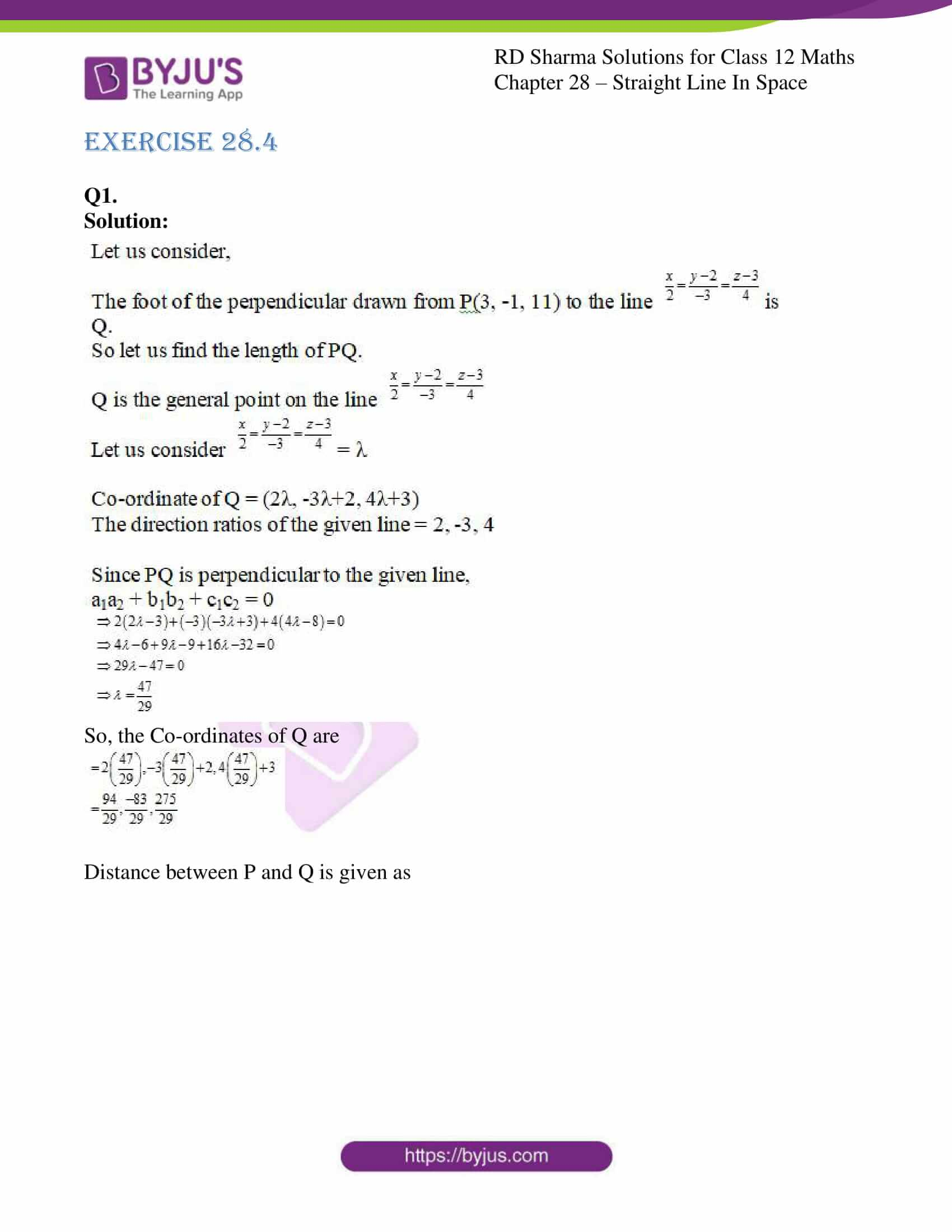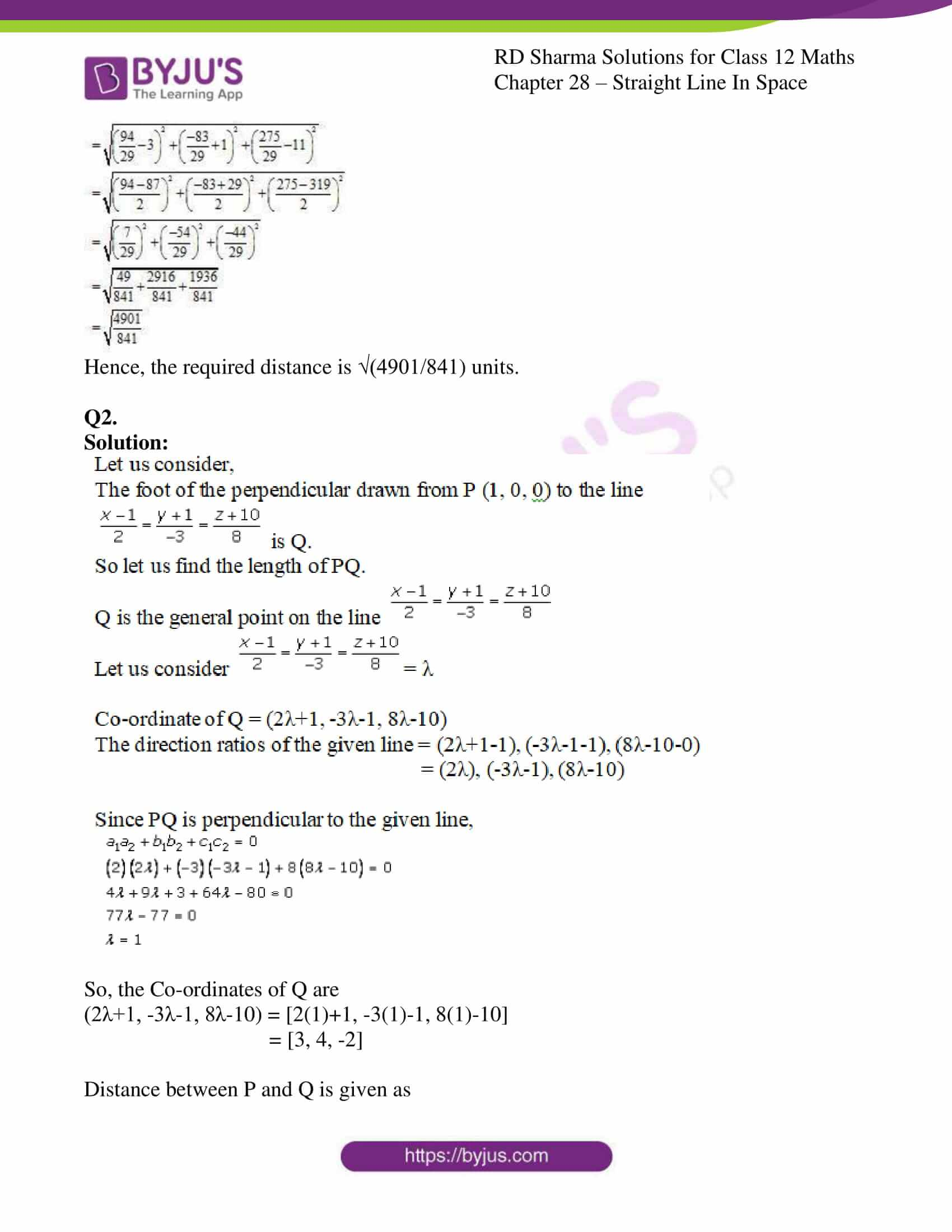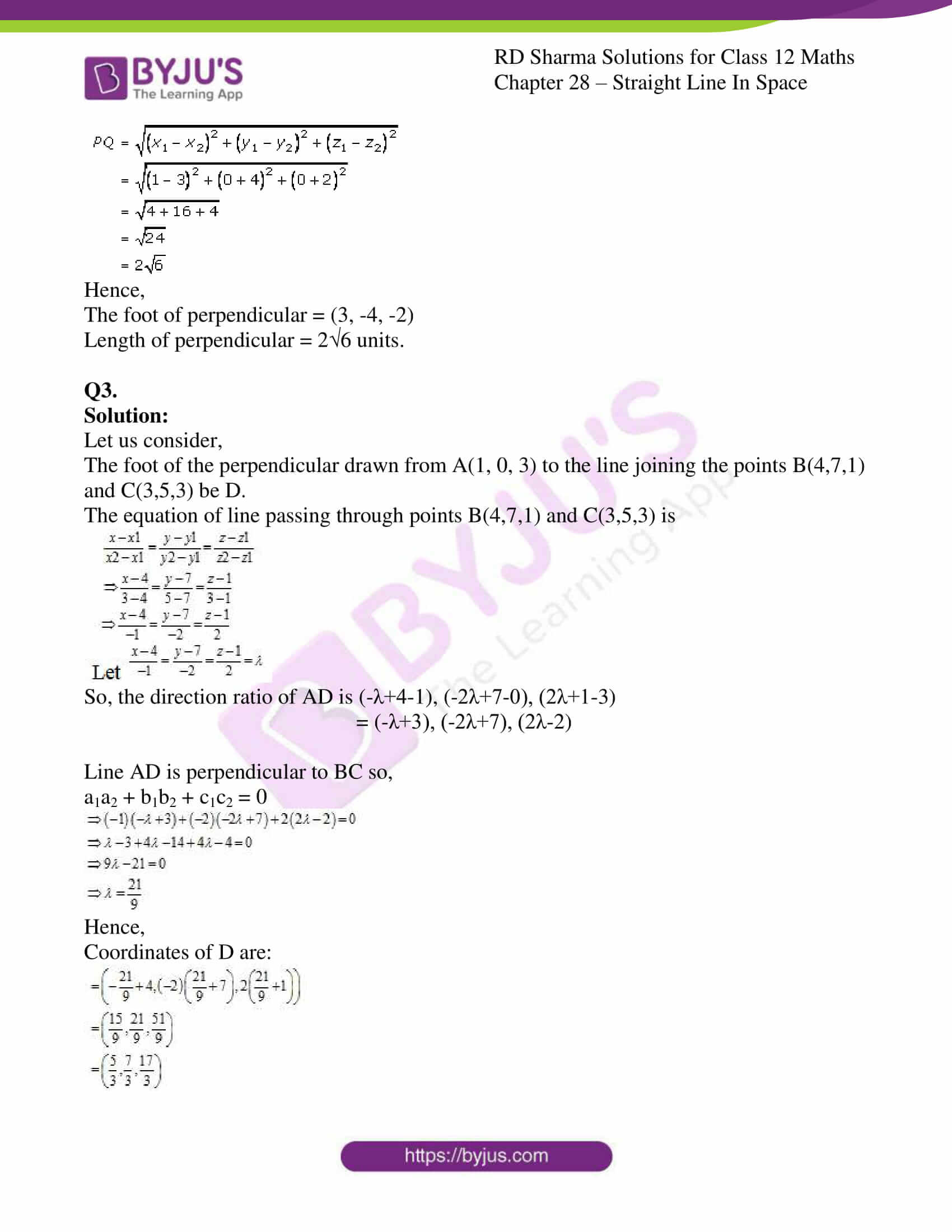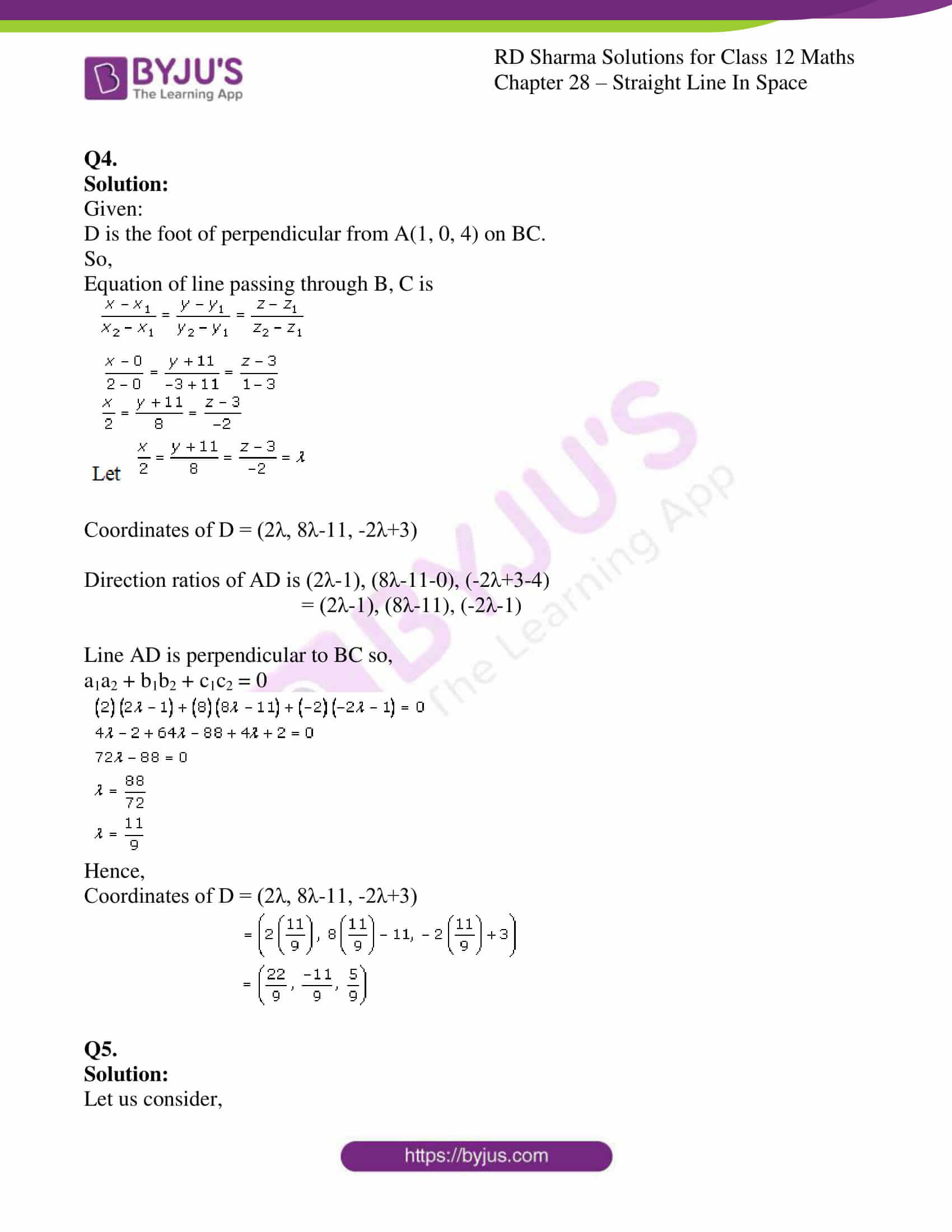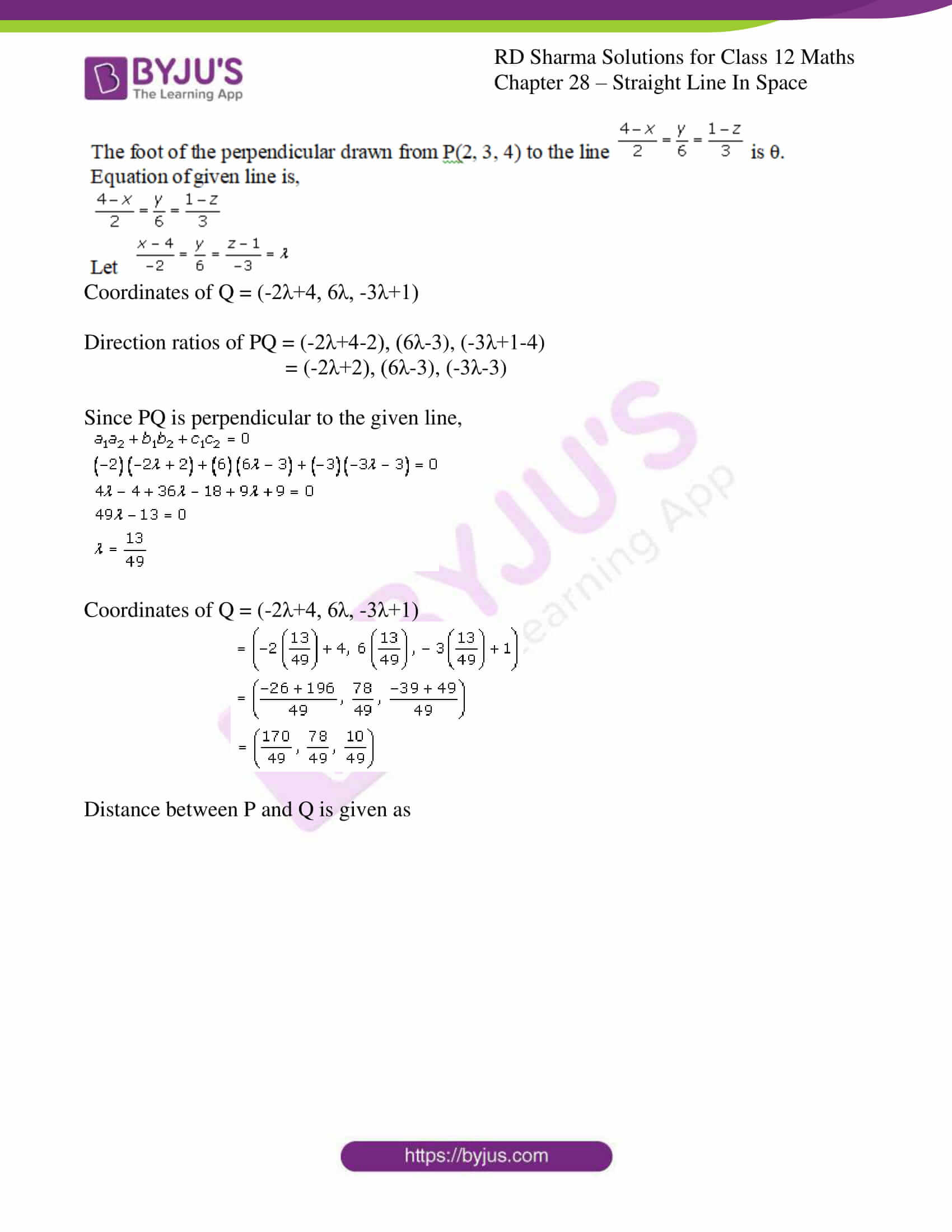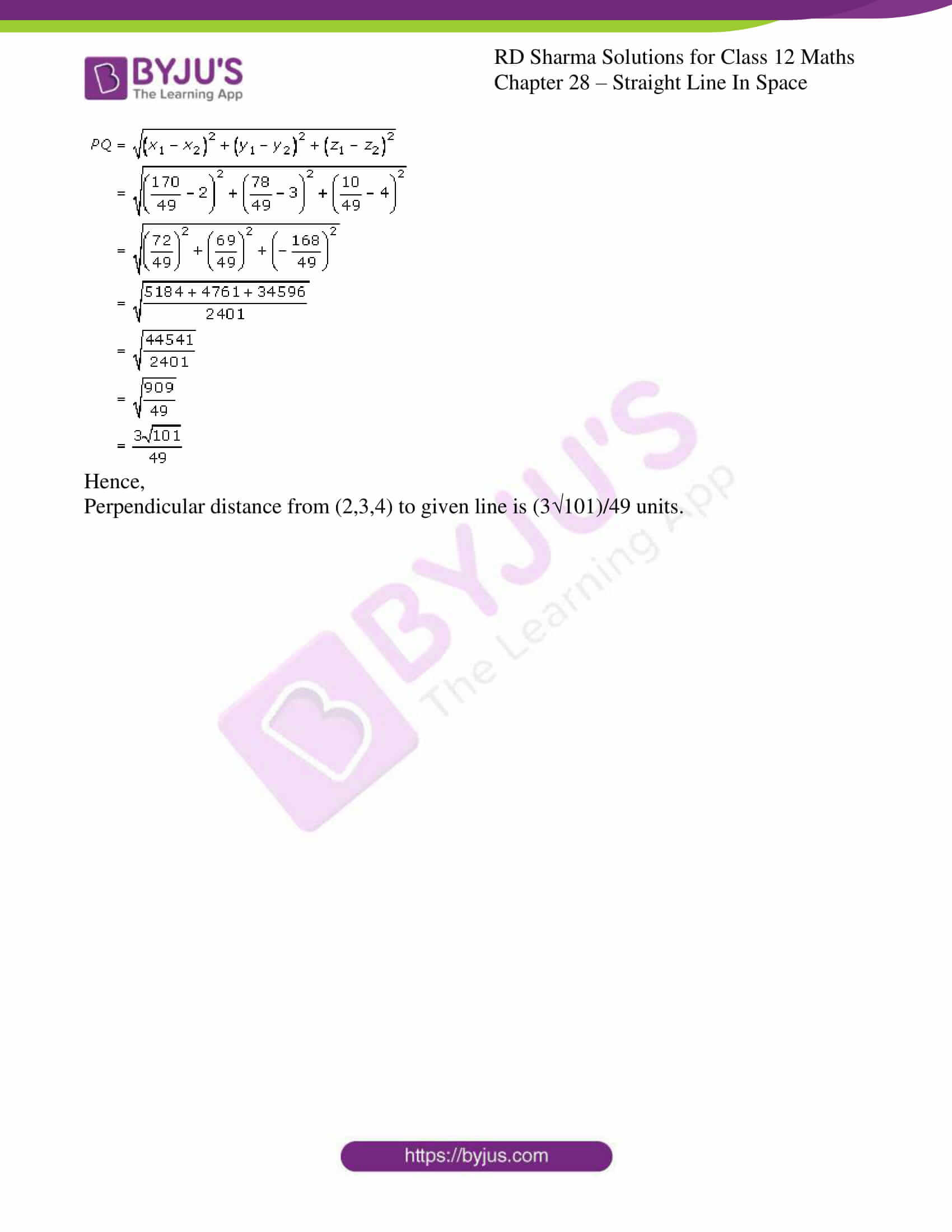### Access Answers for Rd Sharma Solution Class 12 Maths Chapter 28 Exercise 4

Q1.

Solution: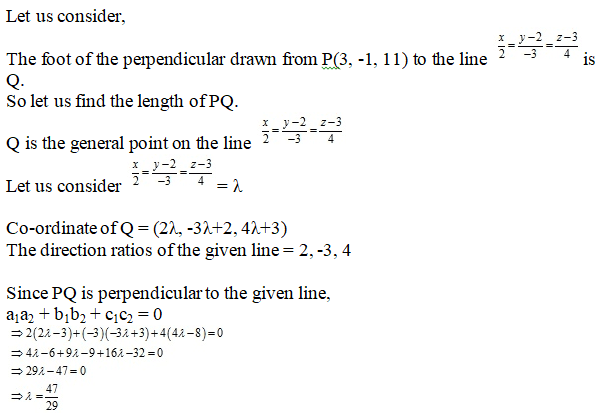So, the Co-ordinates of Q are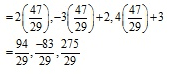Distance between P and Q is given as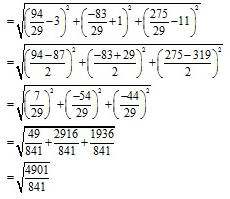Hence, the required distance is √(4901/841) units.

Q2.

Solution: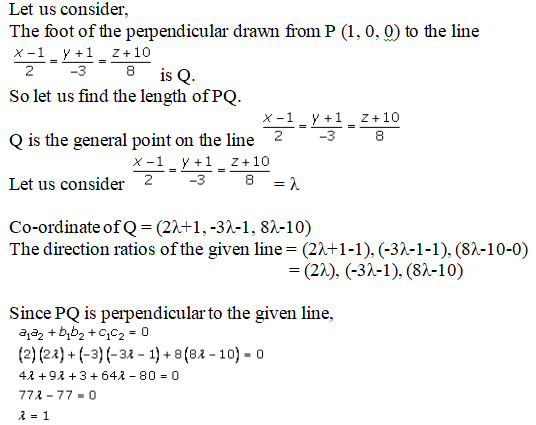So, the Co-ordinates of Q are

(2λ+1, -3λ-1, 8λ-10) = [2(1)+1, -3(1)-1, 8(1)-10]

= [3, 4, -2]

Distance between P and Q is given as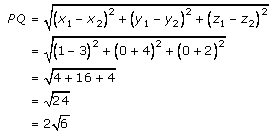Hence,

The foot of perpendicular = (3, -4, -2)

Length of perpendicular = 2√6 units.

Q3.

Solution:

Let us consider,

The foot of the perpendicular drawn from A(1, 0, 3) to the line joining the points B(4,7,1) and C(3,5,3) be D.

The equation of line passing through points B(4,7,1) and C(3,5,3) is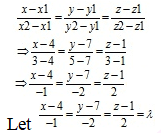So, the direction ratio of AD is (-λ+4-1), (-2λ+7-0), (2λ+1-3)

= (-λ+3), (-2λ+7), (2λ-2)

Line AD is perpendicular to BC so,

a1a2 + b1b2 + c1c2 = 0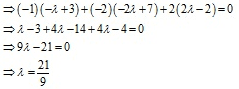Hence,

Coordinates of D are: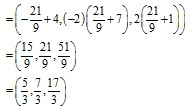Q4.

Solution:

Given:

D is the foot of perpendicular from A(1, 0, 4) on BC.

So,

Equation of line passing through B, C is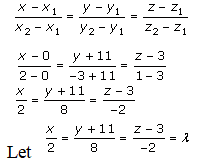Coordinates of D = (2λ, 8λ-11, -2λ+3)

Direction ratios of AD is (2λ-1), (8λ-11-0), (-2λ+3-4)

= (2λ-1), (8λ-11), (-2λ-1)

Line AD is perpendicular to BC so,

a1a2 + b1b2 + c1c2 = 0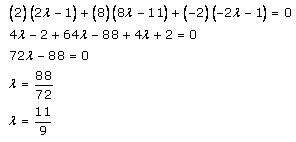Hence,

Coordinates of D = (2λ, 8λ-11, -2λ+3)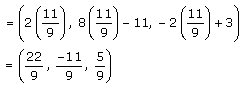Q5.

Solution:

Let us consider,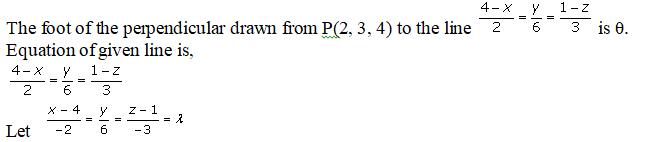Coordinates of Q = (-2λ+4, 6λ, -3λ+1)

Direction ratios of PQ = (-2λ+4-2), (6λ-3), (-3λ+1-4)

= (-2λ+2), (6λ-3), (-3λ-3)

Since PQ is perpendicular to the given line,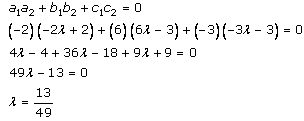Coordinates of Q = (-2λ+4, 6λ, -3λ+1)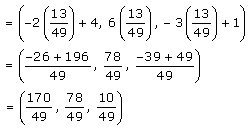Distance between P and Q is given as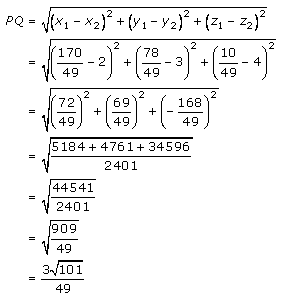Hence,

Perpendicular distance from (2,3,4) to given line is (3√101)/49 units.